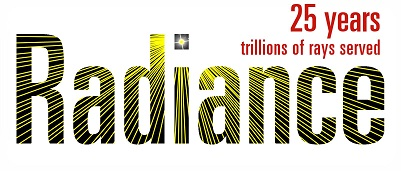# How to properly define the material for a prismatic film in Radiance?

I am working on generating BSDF using genBSDF, and my workflow is shown below:
Firstly, develop the geometry in rhino as shown below:

Secondly, save the geometry model as obj format file, then use obj2rad to convert it to a Radiance model.
Finally, define the material for the radiance model and then use genBSDF to generate the BSDF.

However, to my confusion is the definition of the material. I use dielectric to define the material for the surface, but what is the definition of transmissivity for a closed surface model. For example, for glass, we only use a surface to model it rather than a closed box in Radiance, then define a measured transmissivity in material for a glass of a certain thickness. However, what does transmissivity mean for an interface if we develop a closed surface model? In another word, how to properly determine the transmissitivity for a closed surface model (volume model)?

Hi Yongqing,

Dielectric (and interface) materials use bulk transmissivity per unit length and index of refraction to compute system transmittance. One simple way to compute transmissivity from overall transmittance is to plug the latter with the index of refraction for a known thickness of the material (a plate rather than a prism) and apply the formula in “ray/src/cal/cal/trans.cal”. The following command should work:

rcalc -f trans.cal -e ‘n=\$1;thick=\$2;Tn=\$3;’ -e ‘\$1=tn^(1/thick)’

(Replace single-quotes with double-quotes if using Windows.) Then, give the index of refraction, thickness, index of refraction, and transmittance of the system on the input. E.g., the following input gets the output shown:

1.52 0.06 0.88 [input]
0.492678173 [output]

This is telling you that a system with index of refraction 1.52 (i.e., glass) that is 0.06 world units thick and has a 0.88 (88%) overall transmittance has a transmissivity of 0.492 per unit distance.

Hope this helps!
-Greg

P.S. This may be applied per color channel, giving different transmittance inputs to get the RGB transmissivities if you have that data.